# JP5160980B2 - Vibration suppression method and apparatus - Google Patents

## Info

Publication number
JP5160980B2
JP5160980B2 JP2008178300A JP2008178300A JP5160980B2 JP 5160980 B2 JP5160980 B2 JP 5160980B2 JP 2008178300 A JP2008178300 A JP 2008178300A JP 2008178300 A JP2008178300 A JP 2008178300A JP 5160980 B2 JP5160980 B2 JP 5160980B2
Authority
JP
Japan
Prior art keywords
value
vibration
rotational speed
frequency
rotating shaft
Prior art date
Legal status (The legal status is an assumption and is not a legal conclusion. Google has not performed a legal analysis and makes no representation as to the accuracy of the status listed.)
Active
Application number
JP2008178300A
Other languages
Japanese (ja)
Other versions
JP2010017783A (en
JP2010017783A5 (en
Inventor

Original Assignee
オークマ株式会社
Priority date (The priority date is an assumption and is not a legal conclusion. Google has not performed a legal analysis and makes no representation as to the accuracy of the date listed.)
Filing date
Publication date
Application filed by オークマ株式会社 filed Critical オークマ株式会社
Priority to JP2008178300A priority Critical patent/JP5160980B2/en
Priority claimed from US12/493,494 external-priority patent/US8005574B2/en
Publication of JP2010017783A publication Critical patent/JP2010017783A/en
Publication of JP2010017783A5 publication Critical patent/JP2010017783A5/ja
Application granted granted Critical
Publication of JP5160980B2 publication Critical patent/JP5160980B2/en
Application status is Active legal-status Critical
Anticipated expiration legal-status Critical

## Images

•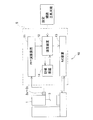•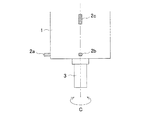•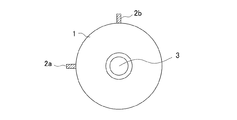•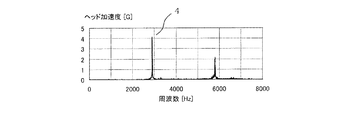•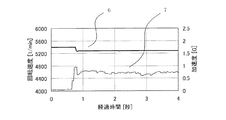•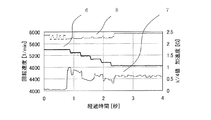•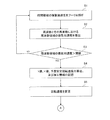•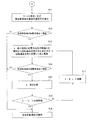## Description

The present invention relates to a method for suppressing vibration generated during processing in a machine tool that performs processing while rotating a tool or a workpiece, and a vibration suppression device capable of executing the method.

Conventionally, for example, a method described in Patent Document 1 is known as a vibration suppression method for machine tools. In this vibration suppression method, in order to suppress regenerative chatter vibration as self-excited vibration that causes deterioration of the finishing accuracy of the machined surface, a natural frequency of a system in which chatter vibration such as a tool or a work is generated is obtained, The value obtained by multiplying and dividing by the number of tool blades and a predetermined integer is taken as the stable rotation speed. And it is going to suppress the chatter vibration which generate | occur | produces during a process by processing at the said stable rotational speed. The natural frequency is obtained by impulse vibration of a tool or workpiece.

A vibration suppression method described in Patent Document 2 is also known. In this vibration suppression method, chatter vibration during machining of a system in which chatter vibration occurs is obtained, and this is multiplied by 60 and machining is performed with a value obtained by dividing the number of tool blades and a predetermined integer as a stable rotation speed, thereby causing chatter vibration. It is intended to suppress. The chatter frequency during machining is obtained based on the vibration frequency detected by the sound sensor during rotation by arranging a sound sensor near the tool or workpiece.

JP 2003-340627 A JP-T-2001-517557

However, the vibration suppression method described in Patent Document 1 requires an expensive impulse device, and the vibration using this device requires a high level of technology and is troublesome. In addition, since the natural frequency obtained before machining does not always match the chatter frequency generated during machining, there is a problem that it is difficult to obtain an accurate stable rotational speed.
On the other hand, in the vibration suppression method described in Patent Document 2, the chatter frequency obtained by analyzing the rotational sound and the chatter frequency actually generated (natural frequency) are slightly different from each other. After all, it is difficult to obtain an accurate stable rotation speed. For this reason, the present applicant installs a detecting means for detecting the vibration in the time domain of the rotating rotating shaft and a calculating means for calculating the chatter frequency based on the vibration in the time domain, and more accurately. A vibration suppression device (for example, Japanese Patent Application No. 2007-138166) was devised to obtain the chatter frequency and to obtain an optimum stable rotation speed. However, in the vibration suppression device, due to the detection error of the detection means, a calculation error occurs between the chatter frequency calculated by the calculation means and the chatter frequency actually generated, and the rotating shaft rotates stably. It is possible that chatter vibration will continue despite the speed.

Therefore, the present invention has been made in view of the above problems, and in the case where chatter vibration continues, a more accurate stable rotational speed can be obtained, and vibration that can reliably suppress chatter vibration. It is an object of the present invention to provide a suppression method and apparatus.

In order to achieve the above object, the invention according to claim 1 of the present invention is a chatter vibration generated when a rotating shaft for rotating a tool or a workpiece is rotated in the machine tool. A vibration suppression method for suppressing vibration, wherein a first step of detecting vibration in the time domain due to the rotating shaft during rotation, and a chatter frequency and a frequency domain in the chatter frequency based on the detected vibration in the time domain When the vibration acceleration of the calculated frequency domain exceeds a predetermined threshold value, the k value and the k ′ value are calculated by the following arithmetic expressions (1) and (2), The third step stored as machining information and the calculated k value are used to calculate the expected stable rotational speed by the following calculation formula (3), and the fourth step is set to the predicted stable rotational speed. And when the vibration acceleration in the frequency domain again exceeds a predetermined threshold value on the rotating shaft that is rotating at the predicted stable rotation speed, the rotation speed of the rotating shaft is changed from the predicted stable rotation speed. And a sixth step of calculating a k ″ value by the same arithmetic expression as the arithmetic expression (1) using the rotation speed after the change when the vibration acceleration in the frequency domain exceeds a predetermined threshold, and the calculated k The change amount, which is the difference between the value and the k ′ value stored as the processing information, is compared with a predetermined phase threshold value. If the change amount does not exceed the phase threshold value, the k ”value is set to the k ′ value. When the change amount exceeds the phase threshold value, the seventh step is updated and the rotation speed change, k ″ value calculation, and k ′ value update are repeated until the change amount exceeds the phase threshold value. To maintain the rotation speed of the It is characterized in that to perform the steps.
Formula (1): k ′ value = {60 × chatter frequency / (number of tool blades × rotational speed)}
Operational expression (2): k value = integer part expression of k ′ value (3): expected stable rotation speed = 60 × chat frequency / {(number of tool blades × (k value + 1)}
Moreover, in order to achieve the said objective, invention of Claim 2 among this invention arises when the said rotating shaft is rotated in the machine tool provided with the rotating shaft for rotating a tool or a workpiece | work. A vibration suppression method for suppressing chatter vibration, the first step of detecting vibration in the time domain due to the rotating shaft during rotation, and the chatter frequency and the chatter frequency based on the detected vibration in the time domain The second step of calculating the vibration acceleration in the frequency domain, and when the calculated vibration acceleration in the frequency domain exceeds a predetermined threshold, the k value and the k ′ value are calculated by the following arithmetic expressions (1) and (2). The third step of storing the k value and k ′ value and the chatter frequency when the vibration acceleration in the frequency domain exceeds a predetermined threshold value as machining information, and using the calculated k value, the following arithmetic expression: ( ) To calculate the expected stable rotational speed, and the fourth step of setting the rotational speed of the rotary shaft as the predicted stable rotational speed, and the vibration acceleration in the frequency domain again on the rotary shaft rotating at the expected stable rotational speed. The fifth step of changing the rotational speed of the rotating shaft from the predicted stable rotational speed when a predetermined threshold is exceeded, and the chatter frequency of the current time and the machining information when the vibration acceleration in the frequency domain exceeds a predetermined threshold The amount of change with the chatter frequency stored as is obtained, the rotational speed is changed until the change exceeds a predetermined second threshold, and the rotational speed at which the change exceeds the predetermined second threshold is determined as the stable rotational speed. And a sixth step maintained as follows.
Formula (1): k ′ value = {60 × chatter frequency / (number of tool blades × rotational speed)}
Arithmetic expression (2): k value = integer part of k ′ value
Formula (3): Expected stable rotational speed = 60 × chat frequency / {(number of tool blades × (k value + 1)}
The “vibration” detected by the first step according to claims 1 and 2 refers to vibration acceleration, displacement due to vibration, sound pressure due to vibration, etc. It includes physical changes that occur and can indirectly detect vibrations.

According to a third aspect of the invention, in the invention of the first or second aspect , when changing the rotation speed in the fifth step, the decimal part of the k ′ value is compared with a predetermined change direction determination threshold value. Further, the increase / decrease of the rotational speed is determined.

On the other hand, the invention according to claim 4 is a vibration suppressing device for suppressing chatter vibration generated when the rotating shaft is rotated in a machine tool including a rotating shaft for rotating a tool or a workpiece. Detecting means for detecting vibration in the time domain of the rotating shaft during rotation, and first calculating means for calculating chatter frequency and vibration acceleration in the frequency domain at the chatter frequency based on the detected vibration in the time domain; When the calculated vibration acceleration in the frequency domain exceeds a predetermined threshold value, the k value and k ′ value are calculated by the following calculation formulas (1) and (2), and the predicted stable rotation speed is calculated by the following calculation formula (3). a second calculating means for respectively calculating a storage means for storing the k value and the k 'value as processing information, and a rotational speed control means for controlling the rotational speed of the rotary shaft, the second arithmetic By means In the rotary shaft during rotation in the issued expected stable rotational speed, again when the vibration acceleration in the frequency domain exceeds a predetermined threshold value, the rotational speed of the rotary shaft with changing from the expected stable rotational speed, frequency When the vibration acceleration of the region exceeds a predetermined threshold, the k ″ value is calculated by the same calculation formula as the calculation formula (1) using the rotation speed after the change, and is stored as the calculated k ″ value and machining information. A change amount that is a difference between the k ′ value and a predetermined phase threshold value, and if the change amount does not exceed the phase threshold value, the k ″ value is updated as the k ′ value, and the change Until the amount exceeds the phase threshold value, the rotation speed change, k ″ value calculation, and k ′ value update are repeated, and the rotation speed when the change amount exceeds the phase threshold value is maintained as the stable rotation speed. It is characterized by this.
Formula (1): k ′ value = {60 × chatter frequency / (number of tool blades × rotational speed)}
Operational expression (2): k value = integer part expression of k ′ value (3): expected stable rotation speed = 60 × chat frequency / {(number of tool blades × (k value + 1)}
The invention according to claim 5 is a vibration suppressing device for suppressing chatter vibration generated when the rotating shaft is rotated in a machine tool having a rotating shaft for rotating a tool or a workpiece. Detecting means for detecting vibration in the time domain of the rotating shaft during rotation, and first calculating means for calculating chatter frequency and vibration acceleration in the frequency domain at the chatter frequency based on the detected vibration in the time domain; When the calculated vibration acceleration in the frequency domain exceeds a predetermined threshold value, the k value and k ′ value are calculated by the following calculation formulas (1) and (2), and the predicted stable rotation speed is calculated by the following calculation formula (3). Second calculating means for calculating the k value, the k ′ value, and the chatter frequency when the vibration acceleration in the frequency domain exceeds a predetermined threshold, as machining information, Rotational speed A rotational speed control means for controlling, when the vibration acceleration in the frequency domain again exceeds a predetermined threshold on the rotating shaft rotating at the expected stable rotational speed calculated by the second computing means, When the rotational speed of the rotating shaft is changed from the predicted stable rotational speed and the vibration acceleration in the frequency domain exceeds a predetermined threshold, the amount of change between the current chatter frequency and the chatter frequency stored as the machining information The rotational speed is changed until the amount of change exceeds a predetermined second threshold, and the rotational speed at which the amount of change exceeds the predetermined second threshold is maintained as a stable rotational speed. .
Formula (1): k ′ value = {60 × chatter frequency / (number of tool blades × rotational speed)}
Arithmetic expression (2): k value = integer part of k ′ value
Formula (3): Expected stable rotational speed = 60 × chat frequency / {(number of tool blades × (k value + 1)}
The “vibration” detected by the detection means in claims 4 and 5 is the same as the “vibration” described in claims 1 and 2 .

According to the present invention, when “chatter vibration” occurs again on the rotating shaft that is rotating at the predicted stable rotational speed, the rotational speed of the rotating shaft is changed based on the predicted stable rotational speed. The generated “chatter vibration” can be suppressed more effectively than before, and as a result, the quality of the machined surface can be improved and the tool wear can be suppressed.
In addition, a more accurate stable rotational speed can be obtained by determining the stable rotational speed by determining the amount of change in k ′ value and the amount of chatter frequency when the rotational speed is changed and comparing it with a phase threshold value. Therefore, “chatter vibration” can be extremely effectively suppressed.

Hereinafter, a vibration suppressing method and apparatus according to an embodiment of the present invention will be described with reference to the drawings.
FIG. 1 is a block diagram illustrating the vibration suppressing device 10. FIG. 2 is an explanatory view showing the rotary shaft housing 1 to be subjected to vibration suppression from the side, and FIG. 3 is an explanatory view showing the rotary shaft housing 1 from the axial direction.

The vibration suppressing device 10 is for suppressing “chatter vibration” generated in the rotating shaft 3 provided in the rotating shaft housing 1 so as to be rotatable around the C axis, and is a time generated in the rotating rotating shaft 3. Vibration sensors (detection means) 2a to 2c for detecting the vibration acceleration of the region (meaning vibration acceleration on the time axis), and the rotation speed of the rotary shaft 3 based on the detection values by the vibration sensors 2a to 2c And a control device 5 for controlling the control.

As shown in FIGS. 2 and 3, the vibration sensors 2 a to 2 c detect time domain vibration accelerations in the X axis, Y axis, and Z axis directions orthogonal to each other in order to detect time domain vibration acceleration in directions perpendicular to each other. It is attached to the rotary shaft housing 1 in a state where vibration acceleration can be detected.

The control device 5 also includes an FFT operation device 11 that performs Fourier analysis based on vibration acceleration in the time domain detected by the vibration sensors 2a to 2c, and a stable rotation speed based on the value calculated by the FFT operation device 11. Is provided with an arithmetic device 12 that calculates the above, an NC device (rotational speed control means) 13 that controls machining in the rotary shaft housing 1, and a storage device 14 that stores various numerical values calculated by the arithmetic device 12. ing. The NC device 13 monitors the rotational speed of the rotary shaft 3.

Here, the vibration suppressing method of “chatter vibration” by the vibration suppressing apparatus 10 as described above will be described based on the flowcharts of FIGS. 7 and 8.
At the beginning of machining, the control device 5 controls the rotation operation of the rotary shaft 3 based on the flowchart of FIG.
First, the FFT arithmetic unit 11 performs Fourier analysis on the vibration acceleration in the time domain that is constantly detected by the vibration sensors 2a to 2c during the rotation of the rotating shaft 3 (S1), and the maximum acceleration (frequency domain) as shown in FIG. Vibration acceleration) and its frequency 4 (chatter frequency) are constantly calculated (S2). When Fourier analysis is performed on the vibration acceleration in the time domain, a plurality of waveforms as shown in FIG. 4 showing the relationship between the frequency and the vibration acceleration in the cirrus region are obtained. In this embodiment, the vibration in the frequency domain is acquired. The waveform that maximizes the acceleration is used.

Next, the arithmetic device 12 compares the vibration acceleration in the frequency domain calculated by the FFT arithmetic device 11 with a predetermined threshold value (S3), and the vibration acceleration in the frequency region exceeds the predetermined threshold value. In the case (for example, when vibration acceleration in the frequency domain at frequency 4 in FIG. 4 is detected), it is assumed that “chatter vibration” to be suppressed occurs on the rotating shaft 3, and the following expression (1), The k ′ value and the k value are calculated by (2), and in addition to the k ′ value and the k value, the vibration acceleration (that is, the maximum acceleration) and the frequency 4 in the frequency domain are stored in the storage device 14 as machining information (S4). ). Further, the predicted stable rotation speed is calculated by the following equation (3), and is output to the NC device 13 to change the rotation speed of the rotary shaft 3 to the predicted stable rotation speed (S5).

k ′ value = {60 × chat frequency / (number of tool blades × rotational speed)} (1)
k value = integer part of k ′ value (2)
Expected stable rotation speed = 60 × chat frequency / {(number of tool blades × (k value + 1)} (3)
Here, it is assumed that “the number of tool blades” in the calculation formulas (1) and (3) is set in the calculation device 12 in advance. In addition, the “rotation speed” in the calculation formula (1) is the current rotation speed before the predicted stable rotation speed. Furthermore, the chatter frequency is a frequency 4 when “chatter vibration” occurs.

When the rotating shaft 3 is rotated at the predicted stable rotational speed calculated as described above, the vibration acceleration 7 at the chatter frequency (frequency 4) is reduced by about 20% as shown in FIG. Therefore, the vibration acceleration in the frequency domain again exceeds the threshold value despite the fact that the rotation shaft 3 is rotated at the expected stable rotation speed calculated as being able to suppress “chatter vibration”, that is, “chatter vibration”. Can occur intermittently. Therefore, after rotating the rotating shaft 3 at the expected stable rotation speed, the control device 5 controls the rotating operation of the rotating shaft 3 based on the flowchart shown in FIG. In addition, 6 in FIG. 5 has shown the rotational speed.

As described above, the FFT arithmetic unit 11 performs Fourier analysis on the time domain vibration acceleration constantly detected by the vibration sensors 2a to 2c during the rotation of the rotating shaft 3 even during rotation at the expected stable rotation speed, and the maximum acceleration and its acceleration. The calculation with the frequency 4 (chatter frequency) is continued (S11), and the calculation device 12 compares the vibration acceleration in the frequency domain calculated by the FFT calculation device 11 with a predetermined threshold value set in advance (S11). S12). And when the vibration acceleration of the frequency domain exceeding a predetermined threshold value is detected again, the fraction part of k 'value memorize | stored in the memory | storage device 14, and the preset change direction determination threshold value (for example, 0.5) The increase / decrease of the rotational speed of the rotating shaft 3 is determined from the following relationship, and the NC apparatus 13 is instructed to slightly change the rotational speed based on the determination (S13). Then, the NC device 13 slightly changes the rotational speed of the rotary shaft 3 in accordance with a command from the arithmetic device 12. Here, the relationship between the decimal part of the k ′ value and the preset change direction determination threshold is that the rotation speed is decreased when the decimal part of the k ′ value is equal to or greater than the change direction determination threshold, and k 'If the decimal part of the value is less than the change direction determination threshold, the rotational speed is increased. Further, the amount of change when the rotational speed is minutely changed is about several percent (for example, 2%) of the rotational speed.

Further, the vibration suppression device 10 continues the Fourier analysis similar to S11 and S12 by the FFT calculation device 11 and the calculation device 12 and the comparison between the vibration acceleration in the frequency domain and a predetermined threshold even after the minute change of the rotation speed (S14). ). Then, when the vibration acceleration exceeding the predetermined threshold is detected again, the arithmetic device 12 calculates the rotational speed after the minute change, the chatter frequency at the vibration acceleration exceeding the predetermined threshold in S14, and the number of tool blades. The k ″ value is calculated using the same equation as the above equation (1) (S15). From the calculated k ″ value and the k ′ value stored in the storage device 14, the following equation ( 4) Find the amount of change.
Change amount = k ′ value−k ″ value (4)

Further, the arithmetic unit 12 compares the amount of change with a preset phase threshold (for example, 0.4) (S16), and if the amount of change does not exceed the phase threshold, the calculated k ″ value is obtained. After the k 'value is overwritten and updated in the storage device 14 (S17), the process returns to S13 to further change the rotation speed, and the amount of change when the “chatter vibration” does not fall out while continuing monitoring based on Fourier analysis. The above control such as obtaining the above is repeatedly executed.
On the other hand, as a result of the comparison in S16, when the amount of change exceeds the phase threshold, and as a result of minutely changing the rotation speed, “chatter vibration” is not detected (that is, vibration in the frequency domain exceeding the predetermined threshold). If the acceleration is no longer detected), the rotation speed is assumed to be a stable rotation speed, and output to the NC device 13 so as to maintain the rotation speed (S18). Then, the NC device 13 that has received the output relating to the maintenance of the rotational speed from the arithmetic device 12 maintains the rotational speed of the rotating shaft 3 at the rotational speed (that is, maintains the stable rotational speed). Therefore, a stable machining state in which “chatter vibration” is suppressed is maintained.

When machining is performed while minutely changing the rotation speed of the rotating shaft 3 as described above, the vibration acceleration 7 at the chatter frequency can be reduced by about 40% as shown in FIG. In other words, theoretically, the phase is 2π (decimal part of k ′ value = 0) is the rotational speed at which the “regenerative chatter vibration” is minimized, but the phase is 2π due to a calculation error or the like. Even if the rotation speed (expected stable rotation speed) is calculated, the rotation speed is not necessarily a stable rotation speed. Therefore, when the phase changes from near 2π to 0, that is, the rotational speed at which the amount of change or chatter frequency changes greatly is the stable rotational speed at which the “regenerative chatter vibration” can be most suppressed. Makes it possible to obtain an accurate stable rotational speed by minutely changing the rotational speed of the rotary shaft 3 from the expected stable rotational speed.

According to the vibration suppression device 10 and the vibration suppression method using the vibration suppression device 10 as described above, the rotation speed of the rotary shaft 3 is slightly changed based on the predicted stable rotation speed, and the change amount of the k ′ value, etc. is calculated. Therefore, more accurate stable rotation speed can be obtained, and chatter vibration generated during machining can be more effectively suppressed than before, and the quality of the machined surface can be improved. Improvement, suppression of tool wear, and the like can be achieved.

The configuration related to the vibration suppression method and apparatus of the present invention is not limited to the aspect described in the above embodiment, and the configuration related to the detection of chatter frequency and the control of vibration suppression is the gist of the present invention. As long as it does not deviate, it can change suitably as needed.

For example, in the above-described embodiment, when “chatter vibration” is intermittently detected, the rotational speed of the rotating shaft is slightly changed, but the rate of change of the rotational speed at this time is the rotational speed before the change. It is better to change it according to the size, number of tool blades, unique system, etc. That is, when the rotational speed is low, a change amount of about 10 min −1 is effective, but the higher the rotational speed, the wider the stable / unstable region. Since the vibration suppressing effect may not be sufficiently obtained unless it is changed by about%, it can be appropriately changed according to the above conditions.
Further, the various threshold values set in advance in the arithmetic expressions (1) to (4) can be appropriately investigated and determined according to the type of the machine tool (for example, in the above embodiment, the phase threshold value is 0). It is possible to take a numerical value between 2 and 0.6, and it is possible to adopt a different numerical value depending on the size / type of the tool or workpiece. Furthermore, in the above embodiment, when obtaining the amount of change to be compared with the phase threshold, the actual amount of change in the k ′ value is obtained from the difference (calculation equation (4)), but the change rate is obtained by differentiation. The ratio may be compared with the phase threshold as a change amount. In addition, it is also possible to adopt not the amount of change in the k ′ value but the amount of change in the chatter frequency before and after the rotation speed change as a change amount to be compared with a predetermined second threshold value.

In the above embodiment, vibration suppression control is performed using a waveform having a maximum vibration acceleration in the frequency domain among waveforms acquired by Fourier analysis of vibration acceleration in the time domain. The predicted stable rotation speed may be calculated using a plurality of (for example, three) waveforms having higher vibration acceleration values in the region, and the effect of suppressing “chatter vibration” may be further improved.
Furthermore, in the above embodiment, the detection means is a vibration sensor, but instead of this, a detection means capable of detecting the displacement of the rotating shaft and the sound pressure due to vibration can be employed. Furthermore, even when a vibration sensor is used, the vibration on the rotating side (that is, the rotating shaft) is not detected as in the above-described embodiment, but the vibration on the non-rotating side is detected, and the expected stable rotational speed is set. You may make it ask.
In addition, the vibration suppressing device according to the present invention is not limited to a machining center that rotates a tool and can also suppress vibrations of a machine tool such as a lathe that rotates a workpiece, and the installation position of the detection means Needless to say, the number and the like can be appropriately changed according to the type and size of the machine tool.

It is block block explanatory drawing of a vibration suppression apparatus. It is explanatory drawing which showed the rotating shaft housing from the side. It is explanatory drawing which showed the rotating shaft housing from the axial direction. It is explanatory drawing which showed an example of the Fourier-analysis result of the vibration acceleration of a time domain. It is explanatory drawing which showed the change of the vibration acceleration in the chatter frequency at the time of setting the rotational speed of a rotating shaft to the expected stable rotational speed. It is explanatory drawing which showed the change of the vibration acceleration in the chatter frequency at the time of making it change minutely after making the rotational speed of a rotating shaft into an expected stable rotational speed. It is a flowchart figure which concerns on suppression control of chatter vibration. It is a flowchart figure which concerns on suppression control of chatter vibration.

Explanation of symbols

Rotating shaft housing, 2a, 2b, 2c, vibration sensor, 3 rotating shaft, 5 control unit, 10 vibration suppression device, 11 FFT processing device, 12 computing device, 13 ..NC device, 14 ..Storage device.

## Claims (5)

1. In a machine tool provided with a rotating shaft for rotating a tool or a work, a vibration suppressing method for suppressing chatter vibration generated when the rotating shaft is rotated,
A first step of detecting time domain vibration by the rotating shaft during rotation;
A second step of calculating a chatter frequency and a vibration acceleration in the frequency domain at the chatter frequency based on the detected vibration in the time domain;
A third step of calculating a k value and a k ′ value according to the following arithmetic expressions (1) and (2) when the calculated vibration acceleration in the frequency domain exceeds a predetermined threshold, and storing it as machining information;
A fourth step of calculating an expected stable rotational speed using the calculated k value according to the following equation (3), and setting the rotational speed of the rotating shaft to the predicted stable rotational speed;
A fifth step of changing the rotational speed of the rotating shaft from the predicted stable rotational speed when the vibration acceleration in the frequency domain again exceeds a predetermined threshold value on the rotating shaft rotating at the predicted stable rotational speed ;
A sixth step of calculating a k ″ value by the same arithmetic expression as the arithmetic expression (1) using the rotational speed after the change when the vibration acceleration in the frequency domain exceeds a predetermined threshold;
The amount of change, which is the difference between the calculated k ″ value and the k ′ value stored as machining information, is compared with a predetermined phase threshold value. If the amount of change does not exceed the phase threshold value, the k ″ value is a seventh step of updating as the k ′ value;
Until the change amount exceeds the phase threshold value, the rotation speed change, the k ″ value calculation, and the k ′ value update are repeated, and the rotation speed when the change amount exceeds the phase threshold value is set as the stable rotation speed. A vibration suppressing method comprising: performing an maintaining step (8) .
Formula (1): k ′ value = {60 × chatter frequency / (number of tool blades × rotational speed)}
Operational expression (2): k value = integer part expression of k ′ value (3): expected stable rotation speed = 60 × chat frequency / {(number of tool blades × (k value + 1)}
2. In a machine tool provided with a rotating shaft for rotating a tool or a work, a vibration suppressing method for suppressing chatter vibration generated when the rotating shaft is rotated,
A first step of detecting time domain vibration by the rotating shaft during rotation;
A second step of calculating a chatter frequency and a vibration acceleration in the frequency domain at the chatter frequency based on the detected vibration in the time domain;
When the calculated vibration acceleration in the frequency domain exceeds a predetermined threshold, the k value and the k ′ value are calculated by the following arithmetic expressions (1) and (2). A third step of storing the chatter frequency when the vibration acceleration of exceeds a predetermined threshold as machining information;
A fourth step of calculating an expected stable rotational speed using the calculated k value according to the following equation (3), and setting the rotational speed of the rotating shaft to the predicted stable rotational speed;
A fifth step of changing the rotational speed of the rotating shaft from the predicted stable rotational speed when the vibration acceleration in the frequency domain again exceeds a predetermined threshold value on the rotating shaft rotating at the predicted stable rotational speed;
When the vibration acceleration in the frequency domain exceeds a predetermined threshold, the amount of change between the current chatter frequency and the chatter frequency stored as the machining information is obtained, and the rotational speed until the change exceeds a predetermined second threshold. And a sixth step of maintaining the rotational speed at which the amount of change exceeds a predetermined second threshold as a stable rotational speed;
The vibration suppression method characterized by performing.
Formula (1): k ′ value = {60 × chatter frequency / (number of tool blades × rotational speed)}
Arithmetic formula (2): k value = integer part of k ′ value
Formula (3): Expected stable rotational speed = 60 × chat frequency / {(number of tool blades × (k value + 1)}
3. 3. The increase / decrease of the rotation speed is determined by comparing the decimal part of the k ′ value with a predetermined change direction determination threshold when changing the rotation speed in the fifth step. Vibration suppression method.
4. In a machine tool provided with a rotating shaft for rotating a tool or a workpiece, a vibration suppressing device for suppressing chatter vibration generated when the rotating shaft is rotated,
Detecting means for detecting vibration in the time domain of the rotating shaft during rotation;
First operation means for calculating a chatter frequency and vibration acceleration in the frequency domain at the chatter frequency based on the detected vibration in the time domain;
When the calculated vibration acceleration in the frequency domain exceeds a predetermined threshold, the k value and the k ′ value are calculated by the following calculation formulas (1) and (2), and the predicted stable rotation speed is calculated by the following calculation formula (3). A second calculation means for calculating each;
Storage means for storing the k value and k ′ value as machining information;
A rotation speed control means for controlling the rotation speed of the rotation shaft ,
When the vibration acceleration in the frequency domain again exceeds a predetermined threshold value on the rotating shaft that is rotating at the predicted stable rotational speed calculated by the second computing means, the rotational speed of the rotating shaft is converted to the predicted stable rotational speed. with varying from,
When the vibration acceleration in the frequency domain exceeds a predetermined threshold value, the k ″ value is calculated by the same calculation formula as the calculation formula (1) using the rotation speed after the change, and is stored as the calculated k ″ value and machining information. A change amount that is a difference between the k ′ value and a predetermined phase threshold value, and if the change amount does not exceed the phase threshold value, the k ″ value is updated as the k ′ value, and Until the amount of change exceeds the phase threshold value, the rotation speed change, k ″ value calculation, and k ′ value update are repeated, and the rotation speed when the amount of change exceeds the phase threshold value is maintained as a stable rotation speed. A vibration suppressing device characterized by:
Formula (1): k ′ value = {60 × chatter frequency / (number of tool blades × rotational speed)}
Operational expression (2): k value = integer part expression of k ′ value (3): expected stable rotation speed = 60 × chat frequency / {(number of tool blades × (k value + 1)}
5. In a machine tool provided with a rotating shaft for rotating a tool or a workpiece, a vibration suppressing device for suppressing chatter vibration generated when the rotating shaft is rotated,
Detecting means for detecting vibration in the time domain of the rotating shaft during rotation;
First operation means for calculating a chatter frequency and vibration acceleration in the frequency domain at the chatter frequency based on the detected vibration in the time domain;
When the calculated vibration acceleration in the frequency domain exceeds a predetermined threshold, the k value and the k ′ value are calculated by the following calculation formulas (1) and (2), and the predicted stable rotation speed is calculated by the following calculation formula (3). A second calculation means for calculating each;
Storage means for storing the k value and k 'value and chatter frequency when the vibration acceleration in the frequency domain exceeds a predetermined threshold as machining information;
Rotation speed control means for controlling the rotation speed of the rotation shaft;
With
When the vibration acceleration in the frequency domain again exceeds a predetermined threshold value on the rotating shaft that is rotating at the predicted stable rotational speed calculated by the second computing means, the rotational speed of the rotating shaft is converted to the predicted stable rotational speed. And change from
When the vibration acceleration in the frequency domain exceeds a predetermined threshold, the amount of change between the current chatter frequency and the chatter frequency stored as the machining information is obtained, and the rotational speed until the change exceeds a predetermined second threshold. And a rotational speed at which the amount of change exceeds a predetermined second threshold is maintained as a stable rotational speed.
Formula (1): k ′ value = {60 × chatter frequency / (number of tool blades × rotational speed)}
Arithmetic formula (2): k value = integer part of k ′ value
Formula (3): Expected stable rotational speed = 60 × chat frequency / {(number of tool blades × (k value + 1)}
JP2008178300A 2008-07-08 2008-07-08 Vibration suppression method and apparatus Active JP5160980B2 (en)

## Priority Applications (1)

Application Number Priority Date Filing Date Title
JP2008178300A JP5160980B2 (en) 2008-07-08 2008-07-08 Vibration suppression method and apparatus

## Applications Claiming Priority (5)

Application Number Priority Date Filing Date Title
JP2008178300A JP5160980B2 (en) 2008-07-08 2008-07-08 Vibration suppression method and apparatus
US12/493,494 US8005574B2 (en) 2008-07-08 2009-06-29 Vibration suppressing method and device
ITMI2009A001175A IT1396911B1 (en) 2008-07-08 2009-07-02 Method and vibration suppressing device
DE200910032233 DE102009032233A1 (en) 2008-07-08 2009-07-08 Vibration suppression method and device
CN 200910158478 CN101623835B (en) 2008-07-08 2009-07-08 Vibration suppressing method and device

## Publications (3)

Publication Number Publication Date
JP2010017783A JP2010017783A (en) 2010-01-28
JP2010017783A5 JP2010017783A5 (en) 2011-04-14
JP5160980B2 true JP5160980B2 (en) 2013-03-13

# Family

## Family Applications (1)

Application Number Title Priority Date Filing Date
JP2008178300A Active JP5160980B2 (en) 2008-07-08 2008-07-08 Vibration suppression method and apparatus

## Country Status (2)

JP (1) JP5160980B2 (en)
CN (1) CN101623835B (en)

## Families Citing this family (22)

* Cited by examiner, † Cited by third party
Publication number Priority date Publication date Assignee Title
JP5215064B2 (en) * 2008-07-17 2013-06-19 オークマ株式会社 Method and apparatus for suppressing chatter vibration of machine tool
US20110074807A1 (en) 2009-09-30 2011-03-31 Hitachi, Ltd. Method of color customization of content screen
CN101947744A (en) * 2010-08-31 2011-01-19 西安瑞特快速制造工程研究有限公司 Method for monitoring gear box state based on numerical control equipment built-in sensor information
JP5536608B2 (en) 2010-10-13 2014-07-02 オークマ株式会社 Vibration suppressing method and vibration suppressing device for machine tool
US9010453B2 (en) * 2010-10-20 2015-04-21 Okuma Corporation Method for monitoring rotary shaft rotation speed fluctuation in machine tool, monitor apparatus, and machine tool
JP5525411B2 (en) 2010-10-25 2014-06-18 オークマ株式会社 Vibration suppression method and vibration suppression apparatus
JP5631758B2 (en) * 2011-01-19 2014-11-26 オークマ株式会社 Vibration suppression device
CN102284888B (en) * 2011-02-25 2013-01-02 华中科技大学 Online monitoring method for turning stability of digital control machine tool
JP5631779B2 (en) * 2011-03-03 2014-11-26 オークマ株式会社 Vibration suppression method and apparatus for machine tool
JP5742312B2 (en) * 2011-03-10 2015-07-01 株式会社ジェイテクト Chatter vibration detection method
JP2012200844A (en) * 2011-03-28 2012-10-22 Jtekt Corp Method for forming chatter stability limit diagram
JP5767931B2 (en) * 2011-09-29 2015-08-26 オークマ株式会社 Vibration suppression method and vibration suppression device for machine tool
CN103116311B (en) * 2012-12-31 2018-10-12 深圳市配天智造装备股份有限公司 A kind of method and device of the acceleration of adjustment digital control system
CN103203670B (en) * 2013-04-03 2015-04-15 同济大学 Grinding chatter prediction method based on maximum information entropy and directional divergence
CN103419090B (en) * 2013-08-19 2016-08-17 南京康尼精密机械有限公司 The vibrating sensing monitoring device of a kind of lathe and method
CN105204443A (en) * 2014-05-21 2015-12-30 Dmg森精机株式会社 Method Of Calculating Stable Spindle Rotation Number Capable Of Suppressing Chatter Vibration, Method Of Informing The Same, Method Of Controlling Spindle Rotation Number, And Method Of Editing Nc Program, And Apparatus Therefor
CN104493636B (en) * 2014-11-12 2017-02-15 华中科技大学 Metallic cryogenic tempering method for improving milling stability
EP3118593A1 (en) * 2015-07-17 2017-01-18 Siemens Aktiengesellschaft Method and detection system for detecting self-starting vibrations
EP3208036A1 (en) * 2016-02-16 2017-08-23 Siemens Aktiengesellschaft Damping of vibrations for a machine tool
CN105619180B (en) * 2016-04-05 2017-10-17 哈尔滨理工大学 A kind of high-speed milling cutter cutter tooth abrasion otherness detection method under effect of vibration
JP6501815B2 (en) * 2017-04-04 2019-04-17 Dmg森精機株式会社 Spindle speed control device
CN108362483A (en) * 2017-12-11 2018-08-03 中国船舶重工集团公司第七〇九研究所 A kind of monitoring method of pipe-line system and monitoring system

## Family Cites Families (7)

* Cited by examiner, † Cited by third party
Publication number Priority date Publication date Assignee Title
JPS6248242B2 (en) 1980-07-03 1987-10-13 Hitachi Ltd
CN1515382A (en) 2001-12-19 2004-07-28 北京工业大学 Machine cutting flutter on-line intelligent control system
JP2005074568A (en) * 2003-09-01 2005-03-24 Mitsubishi Heavy Ind Ltd Multiple spindle machine and workpiece machining method
JP4703315B2 (en) * 2005-08-12 2011-06-15 オークマ株式会社 Rotational speed calculation device of machining device, chatter vibration evaluation device of machining device, and chatter vibration evaluation method of machining device
JP4901271B2 (en) 2006-04-04 2012-03-21 株式会社森精機製作所 Chatter vibration suppression device for machine tools
JP4582661B2 (en) * 2007-05-24 2010-11-17 オークマ株式会社 Vibration suppressor for machine tools
JP4433422B2 (en) * 2007-05-24 2010-03-17 オークマ株式会社 Vibration suppression device

## Also Published As

Publication number Publication date
JP2010017783A (en) 2010-01-28
CN101623835B (en) 2012-12-12
CN101623835A (en) 2010-01-13

## Similar Documents

Publication Publication Date Title
US6266572B1 (en) Apparatus for generating a numerical control command according to cut resistance value and cut torque value of machining simulation
US6662073B1 (en) Apparatus and method for machining simulation for NC machining
KR101300301B1 (en) Method and device for suppressing chattering of work machine
CN101380719B (en) Vibration suppressing device for machine tool
JP5368232B2 (en) Vibration suppression device
US8374717B2 (en) Vibration suppressing method and vibration suppressing device for machine tool
KR101472890B1 (en) Chatter Vibration Detection Method, Chatter Vibration Avoidance Method, and Machine Tool
CN101310921B (en) Vibration suppressing device and vibration suppressing method for machine tool
JP3822565B2 (en) Servo control device
JP4177028B2 (en) Machining method by small diameter end mill and method for determining machining conditions
EP0098309B1 (en) Numerical control machining system
JP4813618B2 (en) Motor control device with function to estimate inertia and friction simultaneously
WO2006016420A1 (en) Method for machining work
JP4565034B2 (en) Control device and control system for inertia estimation
CN102554685B (en) Vibration suppressing method in lathe and equipment for inhibiting of vibration
US9513619B2 (en) Numerical control device which performs tapping operation by using a main spindle and a feed shaft
DE102013206243A1 (en) Processing vibration suppression method and processing vibration suppression device for a machine tool
CN102821910A (en) Tool trajectory generation device, tool trajectory computation method, and tool trajectory generation program
CN101623835B (en) Vibration suppressing method and device
CN102554692B (en) Vibration suppressing method and equipment for inhibiting of vibration
Ismail et al. Chatter suppression in five-axis machining of flexible parts
US7657356B2 (en) Controller
JP2013240837A5 (en)
JP2004330368A (en) Method for detecting cutting resistance, method for controlling machining by cutting resistance and control device
JP4581096B2 (en) Friction compensation method, friction compensator, and motor control device

## Legal Events

Date Code Title Description
A621 Written request for application examination

Free format text: JAPANESE INTERMEDIATE CODE: A621

Effective date: 20110228

A521 Written amendment

Effective date: 20110228

Free format text: JAPANESE INTERMEDIATE CODE: A523

A131 Notification of reasons for refusal

Effective date: 20120807

Free format text: JAPANESE INTERMEDIATE CODE: A131

A521 Written amendment

Free format text: JAPANESE INTERMEDIATE CODE: A523

Effective date: 20121005

TRDD Decision of grant or rejection written
A01 Written decision to grant a patent or to grant a registration (utility model)

Free format text: JAPANESE INTERMEDIATE CODE: A01

Effective date: 20121113

A61 First payment of annual fees (during grant procedure)

Free format text: JAPANESE INTERMEDIATE CODE: A61

Effective date: 20121213

R150 Certificate of patent (=grant) or registration of utility model

Free format text: JAPANESE INTERMEDIATE CODE: R150

FPAY Renewal fee payment (prs date is renewal date of database)

Free format text: PAYMENT UNTIL: 20151221

Year of fee payment: 3